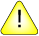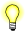This version of this document is no longer maintained. For the latest documentation, see http://www.qnx.com/developers/docs.

# remainder(), remainderf()

Compute the floating point remainder

## Synopsis:

```#include <math.h>

double remainder( double x,
double y );

float remainderf( float x,
float y );```

## Arguments:

x
The numerator of the division.
y
The denominator.

## Library:

libm

Use the -l m option to qcc to link against this library.

## Description:

The remainder() and remainderf() functions return the floating point remainder r = x - ny, where y is nonzero. The value n is the integral value nearest the exact value x/y. When |n - x/y| = ½, the value n is chosen to be even.

The behavior of remainder() is independent of the rounding mode.

## Returns:

The floating point remainder r = x - ny, where y is nonzero.If an error occurs, these functions return 0, but this is also a valid mathematical result. If you want to check for errors, set errno to 0, call the function, and then check errno again. These functions don't change errno if no errors occurred.

## Classification:

Safety:
Cancellation point No
Interrupt handler No
Signal handler No